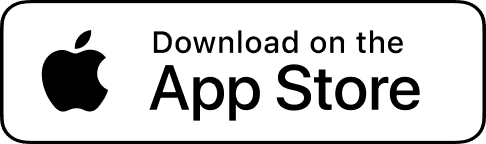Answer.AIWhich formula equation shows a reversible reaction?
a. 2Na+F2–> 2NaF
b. CaCO3–> CaO+CO2
c. NH4CI(s)—–NH3(
g.+HCI(
g. 2H2O2(aq)–pt—> 2H2O(I)+O2(
g.?Solve problem with AIThe formula equation that  shows   a  reversible  reaction  is

NH4Cl (s) ⇔ NH3 (g)  + HCl (g) ( answer  C)

Explanation

• Reversible  reaction  is  a chemical reaction    where the reactants form products , where in turn react together to form reactant   back.
• The  reaction of  NH4Cl (s)⇔ NH3(g)  + HCl (g)  is a reversible reaction  since   NH4Cl  decompose to form NH3  and HCl which in  turn react together to form NH4Cl back .
Recommend videos
You might be interested in...
Explore more...